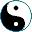<<
>>
 A functor maps one category to another. To do this, we have to map objects of the first category to objects of the second category, and arrows (morphisms) of the first category to arrows of the second category, in a consistent manner. What kind of consistency do we expect? Let X and Y be two categories, and let's start defining a functor F: X → Y. We have to map objects of X to Y, having for each a in X an object F(a) in Y, and for an arrow f in X we have to have an arrow F(f) in Y. For consistency we will need the following: for f: a → b have F(f): F(a) → F(b) - domain and codomain preservation; for ida: a → a have F(ida) = idF(a): F(a) → F(a) - unit preservation; for f:a → b and g:b → c F(g ° f) = F(g) ° F(f) - composition preservation. Functor composition is defined in an obvious way: apply one functor, then another.